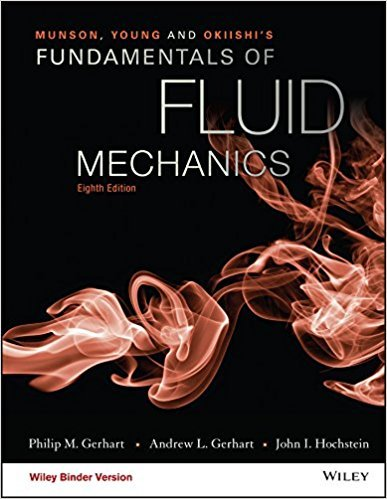×
Get Full Access to Fundamentals Of Fluid Mechanics - 8 Edition - Chapter 5.2.2 - Problem 5.47
Get Full Access to Fundamentals Of Fluid Mechanics - 8 Edition - Chapter 5.2.2 - Problem 5.47

×

# Water flows steadily between fixed vanes, as shown inFig. P5.47. Find the x and yISBN: 9781119080701 456

## Solution for problem 5.47 Chapter 5.2.2

Fundamentals of Fluid Mechanics | 8th Edition

• Textbook Solutions
• 2901 Step-by-step solutions solved by professors and subject experts
• Get 24/7 help from StudySoup virtual teaching assistantsFundamentals of Fluid Mechanics | 8th Edition

4 5 1 333 Reviews
14
2
Problem 5.47

Water flows steadily between fixed vanes, as shown inFig. P5.47. Find the x and y components of the waters forceon the vanes. The total volume flow rate is 100 m3/s, pressurep1 =150kPa, and pressure p2 = 101 kPa.

Step-by-Step Solution:

Step 1 of 3

According to Bernoulli’s equation, the inlet of fluid will be equal to the outlet of fluid on the vanes.For,,,in equation (1).Step 2 of 3

Step 3 of 3

##### ISBN: 9781119080701

Fundamentals of Fluid Mechanics was written by and is associated to the ISBN: 9781119080701. The answer to “Water flows steadily between fixed vanes, as shown inFig. P5.47. Find the x and y components of the waters forceon the vanes. The total volume flow rate is 100 m3/s, pressurep1 =150kPa, and pressure p2 = 101 kPa.” is broken down into a number of easy to follow steps, and 38 words. This full solution covers the following key subjects: . This expansive textbook survival guide covers 112 chapters, and 1357 solutions. The full step-by-step solution to problem: 5.47 from chapter: 5.2.2 was answered by , our top Science solution expert on 03/16/18, 03:21PM. Since the solution to 5.47 from 5.2.2 chapter was answered, more than 1701 students have viewed the full step-by-step answer. This textbook survival guide was created for the textbook: Fundamentals of Fluid Mechanics, edition: 8.

Unlock Textbook Solution